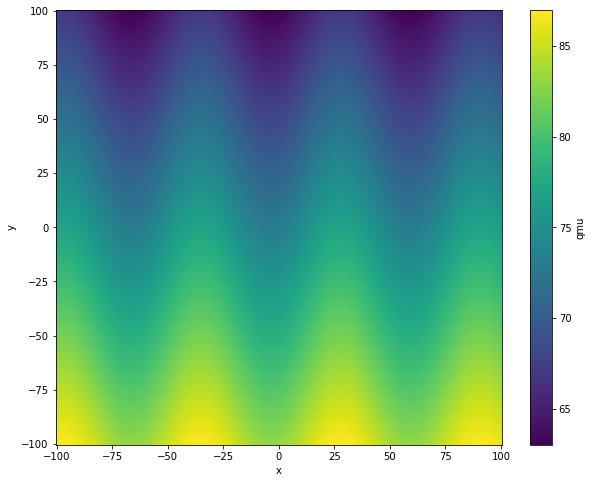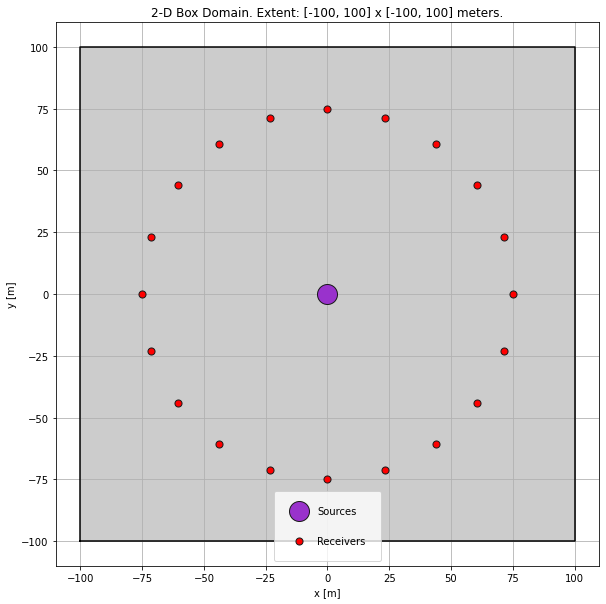Version:

This documentation is not for the latest stable Salvus version.This tutorial is presented as Python code running inside a Jupyter Notebook, the recommended way to use Salvus. To run it yourself you can copy/type each individual cell or directly download the full notebook, including all required files.# Attenuation

This micro-tutorial demonstrates how to run viscoelastic simulations with SalvusProject. It shows the concept in 2-D but it works just the same in 3-D by just adding an extra dimension.
Copy
import matplotlib.pyplot as plt
import numpy as np
import os
import pathlib
import xarray as xr

import salvus.namespace as sn

# Run simulations on this site.
SALVUS_FLOW_SITE_NAME = os.environ.get("SITE_NAME", "local")

## Parameter Model

There are different ways to define meshes and models in SalvusProject. Here we'll define the multi-parameter model as an xarray data set which will later be interpolated onto our numerical meshes.
# Grid points of the velocity model.
# NOT the grid point of the simulation!
nx, ny = 200, 200

# Create a coordinate grid.
x = np.linspace(-100.0, +100.0, nx)
y = np.linspace(-100.0, +100.0, nx)
xx, yy = np.meshgrid(x, y, indexing="ij")

# Simplistic 5 parameter model with a depth gradient and
# some sinusoidal perturbation.
# This is just an example but this would be the place to add
# your own velocity model.
vp = 1500.0 * np.ones_like(xx) - yy * 0.1 + np.sin(xx / 8 + 0.5) * 2
vs = 1000.0 * np.ones_like(xx) - yy * 0.1 + np.sin(xx / 12 - 0.3) * 2
rho = 980.0 * np.ones_like(xx) - yy * 0.1 + np.sin(xx / 15 + 1) * 2

# Assemble into a dataset without Q.
ds_without_q = xr.Dataset(
data_vars={
"vp": (["x", "y"], vp),
"vs": (["x", "y"], vs),
"rho": (["x", "y"], rho),
},
coords={"x": x, "y": y},
)

# Do the same again but this time with a Q model.
qkappa = 2000.0 * np.ones_like(xx) - yy * 0.1 + np.sin(xx / 6) * 2
qmu = 75.0 * np.ones_like(xx) - yy * 0.1 + np.sin(xx / 10 - 1.2) * 2

ds_with_q = xr.Dataset(
data_vars={
"vp": (["x", "y"], vp),
"vs": (["x", "y"], vs),
"rho": (["x", "y"], rho),
"qkappa": (["x", "y"], qkappa),
"qmu": (["x", "y"], qmu),
},
coords={"x": x, "y": y},
)

# Have a look at the Qmu model.
plt.figure(figsize=(10, 8))
ds_with_q.qmu.T.plot()
plt.show()## Create the Project

# Either load an existing project.
if pathlib.Path("project").exists():
print("Opening existing project.")
p = sn.Project(path="project")
# Or create a new one.
else:
print("Creating new project.")
p = sn.Project.from_domain(
path="project",
# Should match the volume model.
domain=sn.domain.dim2.BoxDomain(x0=-100, x1=100, y0=-100, y1=100),
)
Creating new project.


## Simulation Setup

Here we'll create a single explosive source in the center with receivers in a ring around it.
src = sn.simple_config.source.cartesian.MomentTensorPoint2D(
x=0, y=0, mxx=1.0, myy=1.0, mxy=0.0
)

recs = sn.simple_config.receiver.cartesian.collections.RingPoint2D(
x=0, y=0, radius=75.0, count=20, fields=["displacement"]
)

p += sn.Event(event_name="event_a", sources=src, receivers=recs)

p.viz.nb.domain()Now we'll add both velocity models.
p += sn.model.volume.cartesian.GenericModel(
name="model_without_Q", data=ds_without_q
)

p += sn.model.volume.cartesian.GenericModel(
name="model_with_Q", data=ds_with_q
)
# A few wave-lengths are needed to see an attenuating effect.
center_frequency = 100.0

# Set-up two simulations: One with attenuation, one without.
for attenuation in [True, False]:
if attenuation:
simulation_name = "with_attenuation"
mc = sn.ModelConfiguration(
background_model=None,
volume_models="model_with_Q",
# Attenuation results in slightly frequency dependent wave speed.
# Thus one must specify at what frequency they are specified.
# They are often given at 1 Hz.
linear_solids=sn.LinearSolids(reference_frequency=1.0),
)
else:
simulation_name = "without_attenuation"
mc = sn.ModelConfiguration(
background_model=None, volume_models="model_without_Q"
)

p += sn.SimulationConfiguration(
name=simulation_name,
max_frequency_in_hertz=center_frequency * 2.0,
elements_per_wavelength=2.0,
model_configuration=mc,
event_configuration=sn.EventConfiguration(
wavelet=sn.simple_config.stf.Ricker(
center_frequency=center_frequency
),
waveform_simulation_configuration=sn.WaveformSimulationConfiguration(
end_time_in_seconds=0.07,
# This is to tell the simulation to actually
# simulate with attenuation or not.
attenuation=attenuation,
),
),
)

p.viz.nb.simulation_setup("with_attenuation")
[2021-05-27 17:23:34,108] INFO: Creating mesh. Hang on.